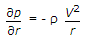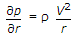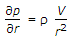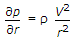# Civil Engineering - Hydraulics

### Exercise :: Hydraulics - Section 6

26.

Flow of water in pipes of diameter more than 3 metres, can be measured by

 A. pitot tube B. venturimeter C. orifice plate D. rotameter.

Explanation:

No answer description available for this question. Let us discuss.

27.

The pressure variation along the radial direction for vortex flow along a horizontal plane is related by

 A.B.C.D.Explanation:

No answer description available for this question. Let us discuss.

28.

 A. remains above the centre line of conduit B. remains below the centre line of conduit C. remains parallel to the centre line of conduit D. may be above or below the centre line of conduit.

Explanation:

No answer description available for this question. Let us discuss.

29.

If the Mach number for a fluid flow is less than 1, the flow is

 A. sonic B. supersonic C. sub-sonic D. none of these.

Explanation:

No answer description available for this question. Let us discuss.

30.

Hydraulic coefficient of an orifice means the coefficient of

 A. velocity B. contraction C. resistance D. all the above.